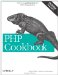# Recipe 2.15. Converting Between Bases

#### 2.15.1. Problem

You need to convert a number from one base to another.

#### 2.15.2. Solution

Use the base_convert( ) function:

`\$hex = 'a1';                           // hexadecimal number (base 16) // convert from base 16 to base 10 \$decimal = base_convert(\$hex, 16, 10); // \$decimal is now 161`

#### 2.15.3. Discussion

The base_convert( ) function changes a string in one base to the correct string in another. It works for all bases from 2 to 36 inclusive, using the letters a through z as additional symbols for bases above 10. The first argument is the number to be converted, followed by the base it is in and the base you want it to become.

There are also a few specialized functions for conversions to and from base 10 and the most commonly used other bases of 2, 8, and 16. They're bindec( ) and decbin( ), octdec( ) and decoct( ), and hexdec( ) and dechex( ) :

`// convert to base 10 print bindec(11011); // 27 print octdec(33);    // 27 print hexdec('1b');  // 27 // convert from base 10 print decbin(27);    // 11011 print decoct(27);    // 33 print dechex(27);    // 1b`

Another alternative is to use printf( ), which allows you to convert decimal numbers to binary, octal, and hexadecimal numbers with a wide range of formatting, such as leading zeros and a choice between upper- and lowercase letters for hexadecimal numbers.

For instance, say you want to print out HTML color values:

`printf('#%02X%02X%02X', 0, 102, 204); // #0066CC`

Documentation on base_convert( ) at http://www.php.net/base-convert and sprintf( ) formatting options at http://www.php.net/sprintf.PHP Cookbook: Solutions and Examples for PHP Programmers
ISBN: 0596101015
EAN: 2147483647
Year: 2006
Pages: 445

Similar book on Amazon